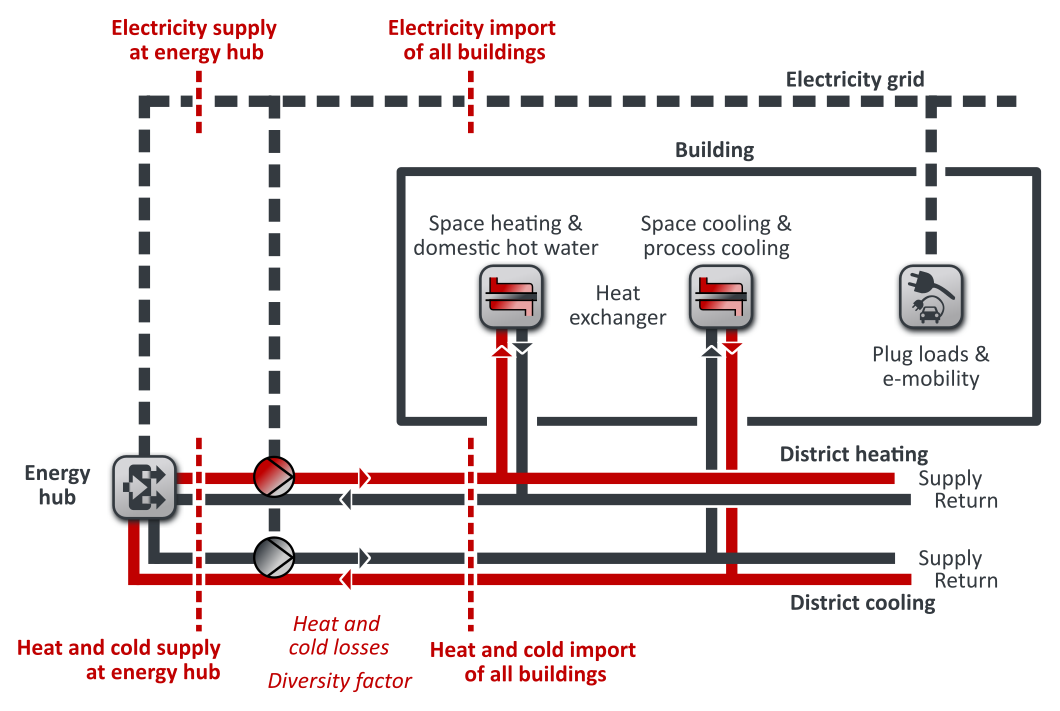The planning tool for district energy systems

# Energy flows in heating and district cooling networks

This page documents the calculation logic for district heating and district cooling networks in nPro.

## Calculation approach

The calculation of district heating and cooling networks in nPro is illustrated in Figure 1: The heat demands for space heating and domestic hot water are covered with a heat exchanger, which is connected with the district heating network. The total heat import of a building is therefore the sum of the space heating and domestic hot water demands. In the same way, the demands for space cooling and process cooling are covered by another heat exchanger that is connected to the district cooling network. Similarly, the total electricity consumption of a building is the sum of the plug loads and electricity demands for e-mobility. The cumulative energy import of all buildings is the sum of heating, cooling and electricity imports of all buildings. When calculating the heat fed into the district heating network by the energy hub, the heat losses of the network and the diversity (simultaneity) factor is taken into account. The heat losses increase the amount of heat to be injected at the energy hub. A simultaneity factor of less than 1 reduces the peak load of the cumulative heat profile of all buildings, leaving the annual total unchanged. The district cooling network is calculated in the same way: The cold to be injected at the energy hub increases due to the losses in the district cooling network and the peak loads decrease due to the diversity factor. For the electricity supplied by the energy hub, the calculation differs: For the electricity network, both losses and diversity factors are not taken into account. Instead, the pumping power for the heating and district cooling network is added to the building demands. The described method is illustrated in Figure 1.Figure 1: Balances in nPro for heating, cooling and electricity supply in districts with conventional heating and district cooling networks.
The calculation logic for 5GDHC networks (anergy networks) is described here.

### nPro Webtool

Plan and optimize district energy systems with nPro